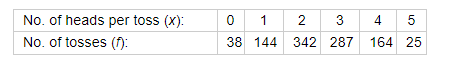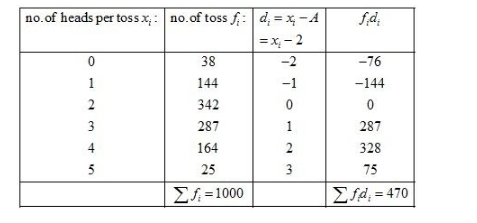# Five coins were simultaneously tossed 1000 times,

Question:

Five coins were simultaneously tossed 1000 times, and at each toss the number of heads was observed. The number of tosses during which 0, 1, 2, 3, 4 and 5 heads were obtained are shown in the table below: Find the mean number of heads per tossSolution:

Let the assume mean be $A=2$.We know that mean $\bar{X}=A+\frac{1}{N} \sum_{i=1}^{n} f_{i} d_{i}$

Now, we have $N=\sum f_{i}=1000, \sum f_{i} d_{i}=470$ and $A=2$

Putting the values above in formula, we have

$\bar{X}=A+\frac{1}{N} \sum_{i=1}^{n} f_{i} d_{i}$

$=2+\frac{1}{1000} \times 470$

$=2+0.47$

$=2.47$

Hence, the mean number of heads per toss is 2.47.